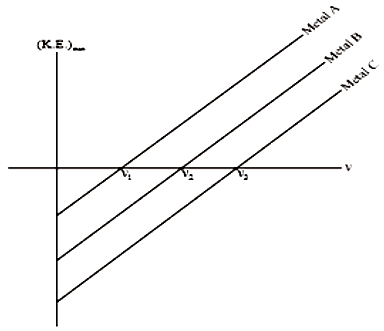For non-relativistic speeds, the wavelength associated with an electron and its kinetic energy E are related as:

1.

2.

3.

4.

Concept Questions :-

Davisson and Germer experiment
High Yielding Test Series + Question Bank - NEET 2020

Difficulty Level:

An electron at rest is acceleration by a potential difference of 600V. The de-Broglie wavelength associated with the electron is:

1.  $1\stackrel{o}{A}$

2.  $2\stackrel{o}{A}$

3.  $3\stackrel{o}{A}$

4.

Concept Questions :-

Davisson and Germer experiment
High Yielding Test Series + Question Bank - NEET 2020

Difficulty Level:

The de-Broglie wavelength of a body of mass 1 kg moving with a velocity of 2000 m/s is

1.

2.

3.

4.  None of these

Concept Questions :-

De-broglie wavelength
High Yielding Test Series + Question Bank - NEET 2020

Difficulty Level:

Light of wavelength 4000 Å falls on a photosensitive metal and a negative 2V potential stops the emitted electrons.

The work function of the material (in eV) is approximately

1.  1.1

2.  2.0

3.  2.2

4.  3.1

Concept Questions :-

Photoelectric effect experiment
High Yielding Test Series + Question Bank - NEET 2020

Difficulty Level:

The work functions for metal A, metal B, and metal C are  respectively. Then-1.

2.

3.

4.  None of these

Concept Questions :-

Einstein photo-electric equation
High Yielding Test Series + Question Bank - NEET 2020

Difficulty Level:

A particle of mass M at rest decays into two particles of

masses having non-zero velocities.

The ratio of de-Broglie wavelength is:

1.

2.

3.  1

4.  $\sqrt{\frac{{\mathrm{m}}_{2}}{{\mathrm{m}}_{1}}}$

Concept Questions :-

De-broglie wavelength
High Yielding Test Series + Question Bank - NEET 2020

Difficulty Level:

If the momentum of a photon is p, then its energy is :

1.  pc

2.

3.

4.  $\frac{1}{4}\mathrm{pc}$

Concept Questions :-

De-broglie wavelength
High Yielding Test Series + Question Bank - NEET 2020

Difficulty Level:

When the light of wavelength  is made to fall on metal in a photoelectric experiment, the maximum kinetic energy of emitted electrons is found to be 2.5eV. The work function of the metal is:

1.  2.5eV

2.  3.1eV

3.  0.9eV

4.  1.5eV

Concept Questions :-

Einstein photo-electric equation
High Yielding Test Series + Question Bank - NEET 2020

Difficulty Level:

The concept of matter-wave was given by:

1.  de-Broglie

2.  Einstein

3.  Maxwell

4.  Bohr

Concept Questions :-

De-broglie wavelength
High Yielding Test Series + Question Bank - NEET 2020

Difficulty Level:

When the light of wavelength $\mathrm{\lambda }$ is used in a photoelectric experiment, the maximum kinetic energy of emitted electrons is found to be K. What will the maximum kinetic energy of the photoelectrons when the light of wavelength $\frac{\mathrm{\lambda }}{2}$ is used instead of $\mathrm{\lambda }$:

1. Zero

2. Non zero but less than 2 K

3. 2K

4. More than 2K

Concept Questions :-

Einstein photo-electric equation